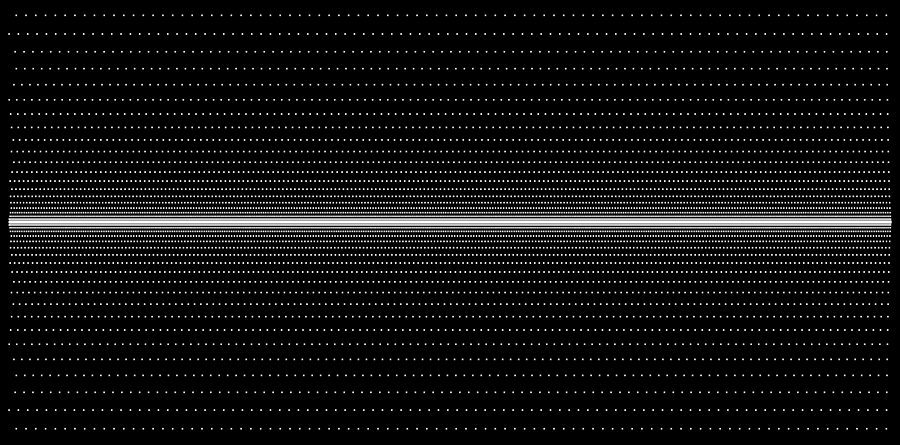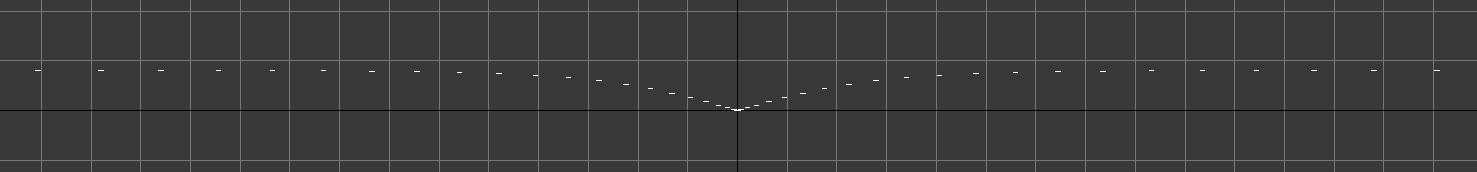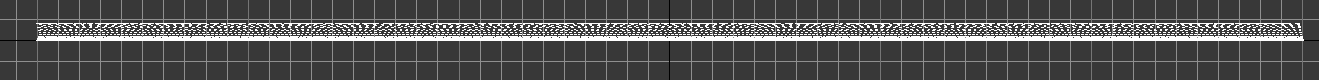# Tiny MAXScript for spreaded boxes at tanh height

This is a tiny MAXScript to create spreaded boxes at the height of a hyperbolic tangent as shown by the images.

20110210-Generator_MAXScript.ms // 1,15 KB (1.181 Bytes)

# Images (Click to view details)

TopFrontRight# Sourcecode

```inputMaxWidth = 600.0
inputMaxHeight = 150.0
inputMaxZ = 8.0
inputIncrease = 0.1
inputIncreaseSave = 0.1

inputCount = 0.0

boxLength = 1.0
boxWidth = 1.0
spaceLine = inputIncrease
widthCount = inputMaxWidth
maxHeight = 0.0
spacing = 0.0
spacingCount = 0.0
currentZAxis = 0.0

-- I'm using while loops here because you can't change the countVar of a for loop.
while maxHeight < inputMaxHeight do (
inputIncrease = inputIncrease + inputIncreaseSave + boxLength / 2
-- Spacing is only counted for the final messageBox:
spacingCount = 0
currentZAxis = 8.0 * tanh(maxHeight/0.5)

while widthCount > 0 do (
boxxy  = box length:boxLength width:boxWidth height:0.01
boxxy.pos = [maxHeight, widthCount, currentZAxis]
widthCount = widthCount - inputIncrease / 2
-- Spacing is only counted for the final messageBox:
spacingCount = spacingCount + 1
)
widthCount = inputMaxWidth - inputIncrease / 4
maxHeight = maxHeight + inputIncrease

)

messageBox ("Done.\nLast spacing value was: " + (inputIncrease as string) +
"\nand the last row counts " + (spacingCount  as string) +
" boxes.\nZ ended at: " + (currentZAxis as string))
```